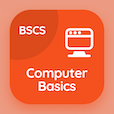Cambridge Online Courses (GCSE)

O Level Physics Quizzes

O Level Physics Quiz PDF - Complete

# Scalar and Vector Multiple Choice Questions p. 104

Study Scalar and Vector multiple choice questions and answers, scalar and vector quiz answers PDF 104 to study O Level Physics course online. Forces in Physics MCQ trivia questions, scalar and vector Multiple Choice Questions (MCQ) for online college degrees. "Scalar and Vector MCQ" PDF eBook: introduction to waves, kinetic molecular model of matter, radiation, boiling and condensation, scalar and vector test prep for two year degree programs.

"Force is a" MCQ PDF: vector quantity, scalar quantity, base quantity, and derived quantity for SAT subject test tutoring. Learn forces in physics questions and answers to improve problem solving skills for two year degree programs.

## Scalar & Vector Questions and Answers MCQs

MCQ: Force is a

Scalar quantity
Vector quantity
Base quantity
Derived quantity

MCQ: Boiling is the change of state

from solid to liquid with change in temperature of 25 °C
from liquid to gas with change in temperature of 75 °C
from solid to liquid without change in temperature
from liquid to gas without change in temperature

MCQ: Good conductor of heat is

Wood
Plastic
Copper
Wool

MCQ: According to kinetic molecular model, in solids,

The particles are closely packed together, they occupy minimum space and are usually arranged in a regular pattern
The particles occur in clusters with molecules slightly further apart
The molecules are very far apart and occupy all the space made available to them
The movement of particles is high speed and random

MCQ: Direction of waves is perpendicular to the direction of vibration in

transverse waves
longitudinal waves
both transverse and longitudinal waves
none of the waves

### More Quizzes from O Level Physics Course